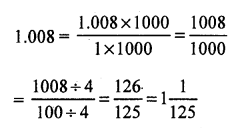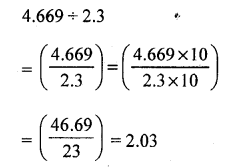# RS Aggarwal Class 7 Solutions Chapter 3 Decimals CCE Test Paper

In this chapter, we provide RS Aggarwal Solutions for Class 7 Chapter 3 Decimals CCE Test Paper for English medium students, Which will very helpful for every student in their exams. Students can download the latest RS Aggarwal Solutions for Chapter 3 Decimals CCE Test Paper Maths pdf, free RS Aggarwal Solutions Chapter 3 Decimals CCE Test Paper Maths book pdf download. Now you will get step by step solution to each question.

### RS Aggarwal Solutions for Class 7 Chapter 3 Decimals CCE Test Paper Download PDF

Question 1.
Solution:
Cost of 1 pen = ₹ 32.50
Cost of 24 such pens = ₹ (32.50 x 24) = ₹ 80
Hence, the cost of 24 pens is ₹ 780.

Question 2.
Solution:
Distance covered by the bus in 1 hour = 64.5 km
Distance covered in 18h = (64.5 x 18) km = 1161 km
Hence, the bus can cover a distance of 1161 km in 18h.

Question 3.
Solution:
First, we will find the product 68 x 65 x 4
Now, 68 x 65 x 4 = 4420 x 4 = 17680
Sum of decimal places in the given decimals = (2 + 1 + 2) = 5
So, the product have five decimal places.
0.68 x 6.5 x 0.04 = 0.17680 = 0.1768

Question 4.
Solution:
Total weight of all the bags = 2231 kg
Weight of each bag = 48.5 kgQuestion 5.
Solution:Question 6.
Solution:
Product of the given decimals = 1.824
One decimal = 0.64
The other decimal = 1.824 ÷ 0.64Hence, the other decimal is 2.85.

Question 7.
Solution:
Thickness of the pile of plywoods = 2.43 m = 2.43 x 100 cm = 243 cm
Thickness of one piece of plywood = 0.45 cm
Required no. of pieces of plywoodHence, the required number of pieces of plywood is 540.

Question 8.
Solution:
Let the number of sides of the polygon be n.
Length of each side of the polygon = 3.8 cm
Perimeter of the polygon = (3.8 x n) cm
But it is given that its perimeter is 22.8 cm.Hence, the given polygon has six sides.

Mark (✓) against the correct answer in each of the following :
Question 9.
Solution:
(b) 2.04Question 10.
Solution:
(b) 11125Question 11.
Solution:
(c) 2.005 kg
2 kg 5 g = (2 x 1000) g + 5 g = (2005)g
= 20051000 kg = 2.005 kg

Question 12.
Solution:
(b) 0.08
We have :Question 13.
Solution:
(c) 0.011
First, we will find the product 11 x 1 x 1
i.e. 11 x 1 x 1 = 11 x 1 = 11
Sum of decimal places in the given decimals = (1 + 1 + 2) = 4
1.1 x 0.1 x 0.01 = 0.0011 [4 places of decimal]

Question 14.
Solution:
(b) 2.03Question 15.
Solution:
(c) is correct
Let the number added be x We have :
2.06 + x = 3.1
⇒ x = 3.1 – 2.06
Converting the given decimals into like decimals, we get:
2.06 and 3.10
Thus, required number = (3.10 – 2.06) = 1.04
Hence, 1.04 should be added to 2.06 to get 3.1.

Question 16.
Solution:
(b) 0.06 .
We have :
0.1 – x = 0.04
⇒ x = 0.1 – 0.04
Converting the given decimals into like decimals, we get:
0.10 and 0.04
Thus, required number = (0.10 – 0.04) = 0.06
Hence, 0.06 should be subtracted from 0.1 to get 0.04

Question 17.
Solution:
(i) 1.001 ÷ 14 = 0.071547 x 53 = 2491
Sum of decimal places in the given decimals = (2 + 1) = 3
0.47 x 5.3 = 2.491
(iv) 0.023 x 0.03 = 0.69
Explanation first, we will multiply 23 by 3
23 x 3 = 69
Sum of decimal places in the given decimals = (3 + 2) = 5
0.023 x 0.03 =0.00069
(v) (0.7)2 = 0.69
Explanation : (0.7)2 = 0.7 x 0.7
First, we will find the product 0.7 x 0.7
Now, 7 x 7 = 49
Sum of decimal places in the given decimals = (1 + 1) = 2
So, the product must have two decimal places.
(0.7)2 = 0.7 x 0.7 = 0.49
(vi) (0.05)3 = 0.000125
Explanation : First, we will find the
product 0.05 x 0.05 x 0.05
Now, 5 x 5 x 5 = 125
Sum of decimal places in the given decimals = (2 + 2 + 2) = 6
So, the product must have six decimal places.
(0.05)2 = 0.05 x 0.05 x 0.05 = 0.000125

Question 18.
Solution:
(i) False
We have :
0.5 x 0.05 Now, 5 x 5 = 25
Sum of decimal places in the given decimals = (1 + 2) = 3
0.5 x 0.5 = 0.025
(ii) True
We have :
0.25 x 0.8
Now, 25 x 8 = 200
Sum of decimal places in the given decimals = (2 + 1) = 3
0.25 x 0.8 = 0.200 = 0.2
(iii) True
We have :
0.35 ÷ 0.7(iv) False We have :
0.4 x 0.4 x 0.4
Now, 4 x 4 x 4 = 64
Sum of decimal places in the given decimals = (1 + 1 + 1) = 3
0.4 x 0.4 x 0.4 = 0.064
(v) True
6 cm = 6100 m = 0.06 m

All Chapter RS Aggarwal Solutions For Class 7 Maths

—————————————————————————–

All Subject NCERT Exemplar Problems Solutions For Class 7

All Subject NCERT Solutions For Class 7

*************************************************

I think you got complete solutions for this chapter. If You have any queries regarding this chapter, please comment on the below section our subject teacher will answer you. We tried our best to give complete solutions so you got good marks in your exam.

If these solutions have helped you, you can also share rsaggarwalsolutions.in to your friends.